Finite Geometry Notes   | Home | Site Map | Author |

## A Four-Color Theorem:Function Decomposition Over a Finite Field by Steven H. Cullinane

The proof of the diamond theorem involves the following elementary, but new and useful, result:

### Every 4-coloring (i.e., every function into a 4-set) can be expressed as a sum of three 2-colorings (into GF(4)).

(Or, equivalently, as a linear combination of three 2-colorings (into GF(2) as a subfield of GF(4))).

How this works:

Let m be a map into a 4-set.

Represent the elements of the 4-set by the elements {0, 1, a, b} of the finite field GF(4), so that m becomes a map f into this field.

Define f(x,y), where x, y are elements of GF(4), as the map into GF(4) that has value 1 wherever f has value x or y, and that has value 0 elsewhere. Then

f = 1f(a,b) + af(1,b) + bf(1,a)
A modest generalization of the decomposition theorem, and a problem disguised as a query, are given by the 1982 research note below.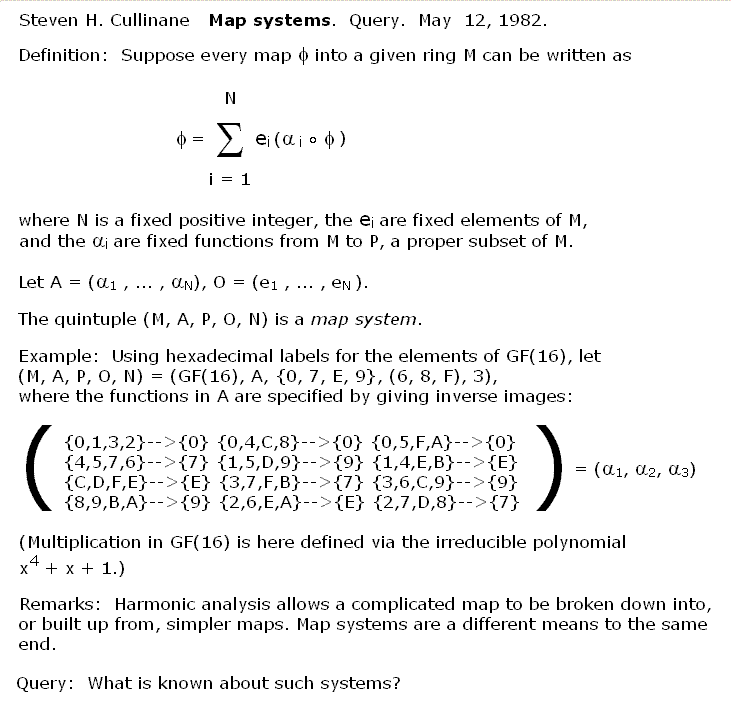View original 1982 note.

The decomposition theorem's algebraic formulation above is useful for showing, for instance, that certain four-colored graphic images can form a ring under multiplication.

But in applying the decomposition theorem, we often are interested only in the partition lines separating regions colored by complementary 2-subsets of the 4-color set.

Example: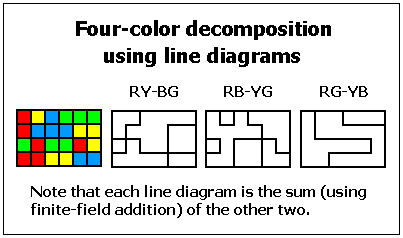Example: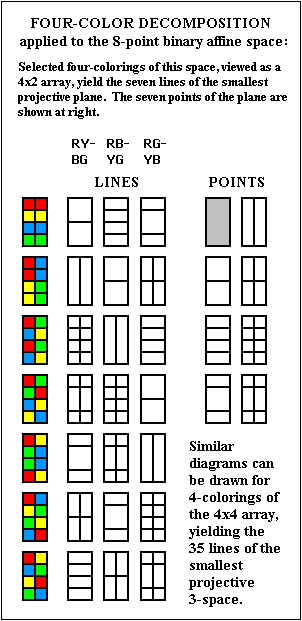Example: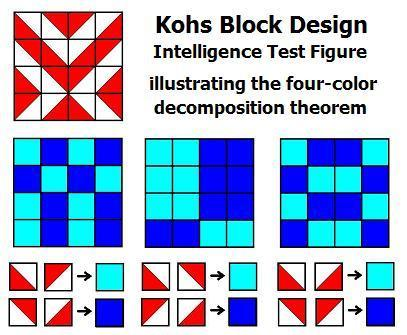Kohs Block Design Test figure
illustrating the four-color decomposition theorem

 Application of Four-Color Decomposition: The Diamond Theorem We regard the four-diamond figure D below as a 4x4 array of two-color diagonally-divided square tiles. Let G be the group of 322,560 permutations of these 16 tiles generated by arbitrarily mixing random permutations of rows and of columns with random permutations of the four 2x2 quadrants. THEOREM: Every G-image of D (as at right, below) has some ordinary or color-interchange symmetry. Example: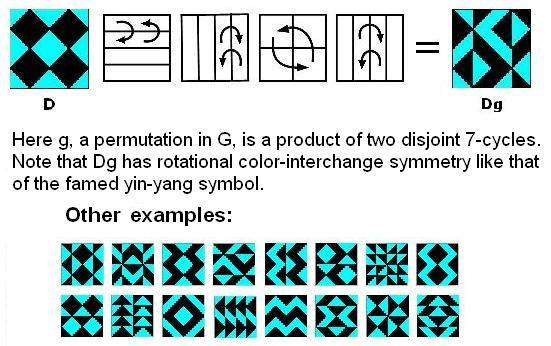For an animated version, click here.

For more on how the decomposition theorem